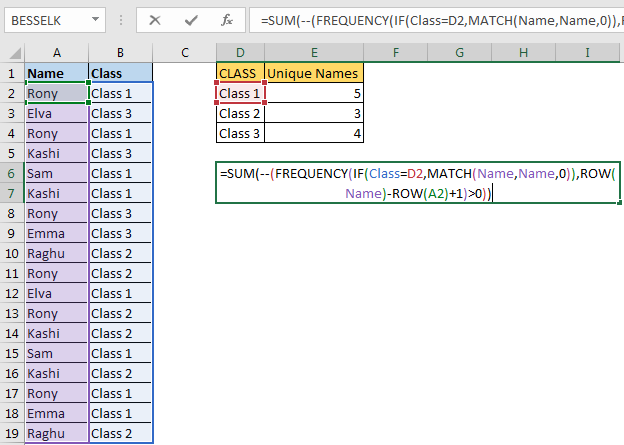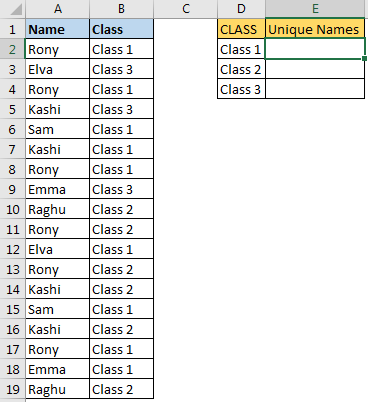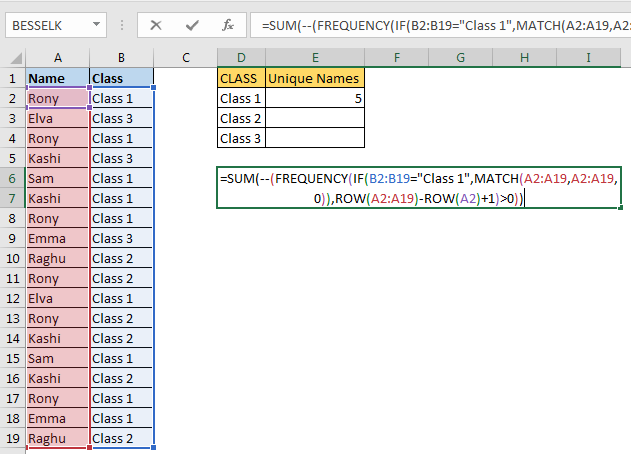# How To Count Unique Values in Excel With Criteria?Earlier we learned how to count unique values in a range. We also learned how to extract unique values from a range. In this article we will learn how to count unique value in range with condition in excel.
Generic Formula

{=SUM(--(FREQUENCY(IF(condition,MATCH(range,range,0)),ROW(range)-ROW(firstCell in range)+1)>0))}

It is an array formula, use CTRL+SHIFT+ENTER

Condition : The criteria on which you want to get unique values.

Range : range in which you want to get unique values.

firstCell in range: It is the reference of the first cell in range. If range is A2:A10 then it is A2.

Example:

Here I have this data of names. The corresponding classes are mentioned in the adjacent column. We need to count unique names in each class.Using above generic formula write this formula in E2

{=SUM(--(FREQUENCY(IF(B2:B19="Class 1",MATCH(A2:A19,A2:A19,0)),ROW(A2:A19)-ROW(A2)+1)>0))}

The above formula returns the unique value in excel range A2:A19 on condition of B2:B19="Class 1".To get unique values in different classes, change the criteria. We have hardcoded it here, but you can give cell reference too. Use named ranges or absolute referencing for ranges, if you don’t want them too change.
How it works?
Let's break it down from inside.

IF(B2:B19="Class 1",MATCH(A2:A19,A2:A19,0))

B2:B19="Class 1": This part will returns an array of true and false. TRUE for each match.

{TRUE;FALSE;TRUE;FALSE;TRUE;TRUE;FALSE….}
MATCH(A2:A19,A2:A19,0): this part will return the first location of each value in range A2:A19 as per MATCH’s property.

{1;2;1;4;5;4;1;8;9;1;2;1;4;5;4;1;8;9}.

Now for each TRUE value we will get the position and for false we will get FALSE. So for whole IF statement we will get

{1;FALSE;1;FALSE;5;4;FALSE;FALSE;FALSE;FALSE;2;FALSE;FALSE;5;FALSE;1;8;FALSE}.

Next we move to frequency part.

FREQUENCY(IF(B2:B19="Class 1",MATCH(A2:A19,A2:A19,0)),ROW(A2:A19)-ROW(A2)+1)
ROW(A2:A19): This returns the row number of each cell in range A2:A19.

{2;3;4;5;6;7;8;9;10;11;12;13;14;15;16;17;18;19}

ROW(A2:A19)-ROW(A2): Now we subtract the first row number from each row number. This returns the an array of serial number starting from 0.

{0;1;2;3;4;5;6;7;8;9;10;11;12;13;14;15;16;17}

Since we want to have serial number starting from 1, we add 1 to it.

ROW(A2:A19)-ROW(A2)+1. This gives us an array of serial number starting from 1.

{1;2;3;4;5;6;7;8;9;10;11;12;13;14;15;16;17;18}

This will help us in getting unique count on condition.

Now we have: FREQUENCY({1;FALSE;1;FALSE;5;4;FALSE;FALSE;FALSE;FALSE;2;FALSE;FALSE;5;FALSE;1;8;FALSE},{1;2;3;4;5;6;7;8;9;10;11;12;13;14;15;16;17;18})

This returns the frequency of each number in given array.{3;1;0;1;2;0;0;1;0;0;0;0;0;0;0;0;0;0;0}

Here each positive number indicated occurrence of unique value when criteria is met. We need to count values greater than 0 in this array. For that we check it by >0. This will return TRUE and FALSE. We convert true false using -- (double binary operator).

SUM(--({3;1;0;1;2;0;0;1;0;0;0;0;0;0;0;0;0;0;0})>0) this translates to SUM({1;1;0;1;1;0;0;1;0;0;0;0;0;0;0;0;0;0;0})

And finally we get the unique count of names in range on criteria as 5.

I know it is bit complex to understand but you check it from formula evaluation option.

To count unique values with multiple criteria we can use boolean logic:

Count Unique Value With Multiple Criterias With And Logic

{=SUM(--(FREQUENCY(IF(condition1 * Condition2,MATCH(range,range,0)),ROW(range)-ROW(firstCell in range)+1)>0))}

Above generic formula can count unique values on multiple conditions and when all of them are true.

Count Unique Value With Multiple Criterias With Or Logic

{=SUM(--(FREQUENCY(IF(condition1 + Condition2,MATCH(range,range,0)),ROW(range)-ROW(firstCell in range)+1)>0))}

This generic formula can be used to count unique values with Or logic. It means, it will count if any of the condition is true.
So yeah guys, this how you count unique values in a range on multiple conditions. This is a little bit complex but it is fast. Once you start using it, you will get how it works.
If you have any doubts regarding this excel formula article then let me know in the comments section below.

Related Articles:

Excel Formula to Extract Unique Values From a List

Count Unique Values In Excel

Popular Articles:

The VLOOKUP Function in Excel

COUNTIF in Excel 2016

How to Use SUMIF Function in Excel

1.